Related Articles

# Pascal’s Triangle

Pascal’s triangle is the arrangement of the data in triangular form which is used to represent the coefficients of the binomial expansions, i.e. the second row in Pascal’s triangle represents the coefficients in (x+y)2 and so on. In Pascal’s triangle, each number is the sum of the above two numbers. Pascal’s triangle has various applications in probability theory, combinatorics, algebra, and various other branches of mathematics.

## What is Pascal’s Triangle?

It is named after the famous Philosopher and Mathematician Balise ‘Pascal’ who developed a pattern of numbers starting with 1 and the numbers beneath are the summation of the above numbers. To start making Pascal’s triangle, first, write down the number 1. The second row is written down by two 1s again. Other rows are generated using the previous rows to make a triangle of numbers. Each row begins and ends with a 1.

A basic structure of the Pascal triangle is shown in the image added below,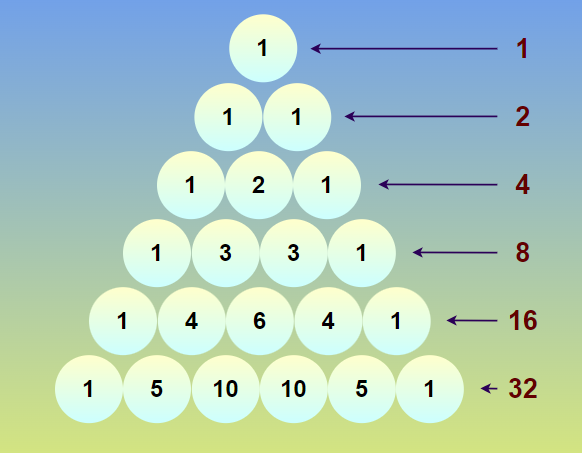### Pascal’s Triangle Definition

We define the Pascal triangle as the basic set of numbers arrange in a triangular array such that each element in Pascal’s triangle is the sum of the two numbers above it. Pascal’s triangle starts with 1  and this was first proposed by the famous French mathematician Balise Pascal and hence named Pascal’s Triangle.

This triangle represents the coefficients of the binomial expansion for various powers. (we have to make sure that the power in the binomial expansion is only a natural number then only Pascal’s triangle represents the coefficients in the binomial expansion)

## Pascal’s Triangle Construction

We can easily construct the Pad=scal’s triangle by just adding the two numbers of the above row to get the next number in the row below. We can assume that the zeroth row starts with a single element 1 and then the element in the second row is 1 1 which is formed by adding 1+0 and 1+0. Similarly, the elements in the second row are, 1 2 1 2hich are formed by adding, 1+0, 1+1, and 1+0, and thus the elements in the third row are obtained. Expanding this concept to the nth row we get a Pascal’s Triangle with n+1 rows.

Pascal’s Triangle upto 3rd row is shown in the image below,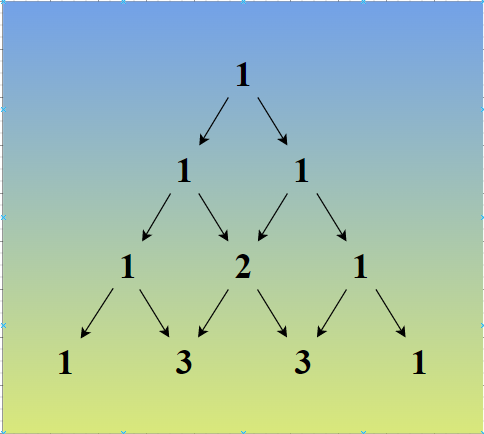From the above figure, we easily observe that the first and the last element in each row is 1.

## Pascal’s Triangle Formula

Pascal Triangle Formula is the formula that is used to find the number that is to be filled in the mth column and the nth row. As we know that the terms in Pascal’s triangle are the summation of the terms in the above row. So we require the elements in the (n-1)th row, and (m-1)th and nth columns to get the required number in the mth column and the nth row.

The elements of the nth row of Pascal’s triangle are given, nC0, nC1, nC2, …, nCn

The formula for finding any number in Pascal’s triangle is:

nCm = n-1Cm-1 + n-1Cm

Where,

• nCm represents the (m+1)th element in the nth row., and
• n is a non-negative integer [0 ≤ m ≤ n]

We can understand this formula using the example discussed below,

Example: Find the third element in the third row of Pascal’s triangle.

Solution:

We have to find the 3rd element in the 3rd row of Pascal’s triangle.

Pascal Triangle Formula is,

nCk = n-1Ck-1  + n-1Ck

where nCk represent (k+1)th element in nth row.

Thus, 3rd element in the 3rd row is,

3C2 = 2C1 + 2C2

3C2 = 2 + 1

3C2 = 3

Thus, the third element in the third row of Pascal’s triangle is 3.

## Pascal’s Triangle Binomial Expansion

We can easily find the coefficient of the binomial expansion using Pascal’s Triangle. The elements in the (n+1)th row of the Pascal triangle represent the coefficient of the expanded expression of the polynomial (x + y)n.

We know that the expansion of (x + y)n is,

(x + y)n = a0xn + a1xn-1y + a2xn-2y2 + … + an-1xyn-1 + anyn

Here, a0, a1, a2, a3, …., an are the term in the (n+1)th row of Pascal’s Triangle

For example, see the expansion of (x+y)4

(x + y)4 = 4C0x4 + 4C1 x3y + 4C2 x2y2 + 4C3 xy3 + 4C4x0y4

⇒ (x + y)4 = (1)x4 + (4)x3y + (6)x2y2 + (4)xy3 + (1)y4

Here, the coefficients 1, 4, 6, 4, and 1 are the elements of the fourth row of Pascal’s Triangle

## How to Use Pascal’s Triangle?

We use the Pascal triangle to find the various case of the possible outcomes in probability conditions. This can be understood by the following example, tossing a coin one time we get two outcomes i.e. H and T this is represented by the element in the first row of Pascal’s Triangle.

Similarly tossing a coin two times we get three outcomes i.e. {H, H}, {H, T}, {T, H}, and {T, T} this condition is represented by the element in the second row of Pascal’s Triangle.

Thus, we can easily tell the possible number of outcomes in tossing a coin experiment by simply observing the respective elements in the Pascal Triangle.

The table below tells us about the cases if a coin is tossed one time, two times, three times, and four times, and its accordance with Pascal’s Triangle

## Pascal’s Triangle Patterns

We observe various patterns in Pascal’s triangle they are:

• Prime Numbers in Triangle
• Diagonals in Pascal’s Triangle
• Fibonacci Pattern

On close observing Pascal’s Triangle we can conclude that the sum of any row in Pascal’s triangle is equal to a power of 2. The formula for the same is, For any (n+1)th row in Pascal’s Triangle the sum of all the elements is, 2n

Applying this Formula in the first 4 rows of Pascal’s triangle we get,

1 = 1 = 20

1 + 1 = 2 = 21

1 + 2 + 1 = 4 = 22

1 + 3 + 3 + 1 = 8 = 23

### Prime Numbers in Pascal’s Triangle

Another very interesting pattern in the Pascals triangle is that if a row starts with a prime number (neglecting 1 at the start of each row), then all the elements in that row are divisible by that prime number. This pattern does not hold true for the composite numbers.

For example, the eighth row in the Pascal triangle is,

1    7    21    35    35    21    7    1

Here, all the elements are divisible by 7.

For rows starting with composite numbers such as the fifth row,

1    4    6    4    1

The pattern does not hold true as 4 does not divide 6.

### Diagonals in Pascal’s Triangle

Each rightward diagonal of Pascal’s Triangle, when considered as a sequence represents the different numbers such as the first rightward diagonal represents a sequence of number 1, the second rightward diagonal represents triangular numbers, the third rightward diagonal represents the tetrahedral numbers, the fourth rightward diagonal represents the Penelope numbers and so on.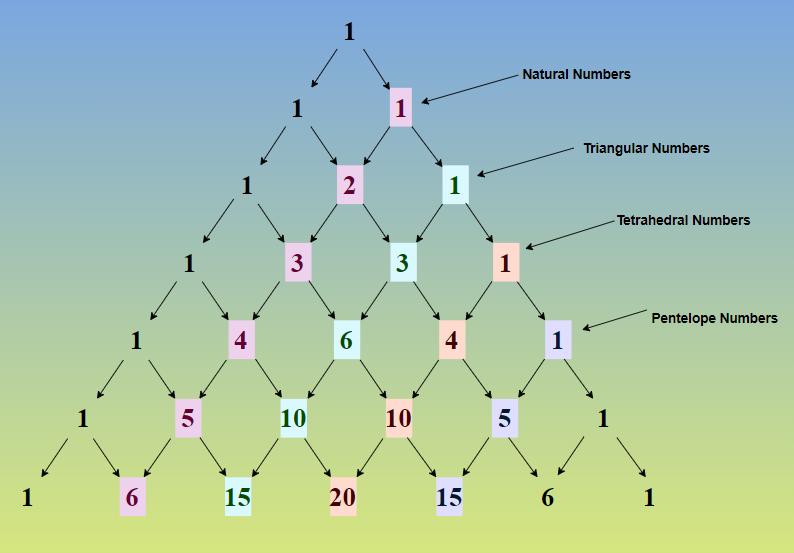### Fibonacci Sequence in Pascal’s Triangle

We can easily obtain the Fibonacci sequence by simply adding the numbers in the diagonals of Pascal’s triangle. This pattern is shown in the image added below,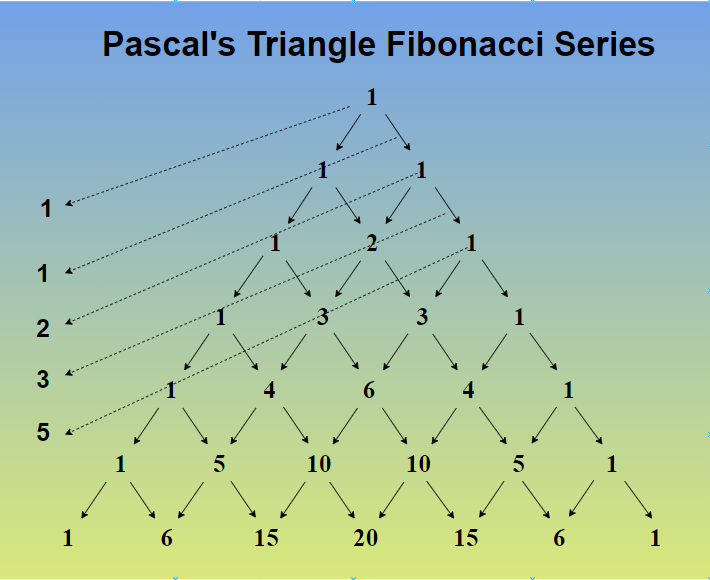## Pascal’s Triangle Properties

Various Properties of Pascal’s Triangle are,

• Every number in the Pascal triangle is the sum of the number above it.
• The starting and the end number in Pascal’s triangle are always 1.
• The first diagonal in Pascal’s Triangle represents the natural number or counting numbers.
• The sum of elements in each row of Pascal’s triangle is given using a power of 2.
• Elements in each row are the digits of the power of 11.
• The Pascal triangle is a symmetric triangle.
• The elements in any row of Pascal’s triangle can be used to represent the coefficients of Binomial Expansion.
• Along the diagonal of Pascal’s Triangle, we observe the Fibonacci numbers.

## Pascal’s Triangle Examples

Example 1: Find the fifth row of Pascal’s triangle.

Solution:

The Pascal triangle with 5 row is shown in the image below,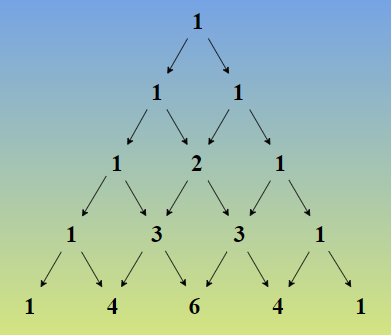Example 2: Expand using Pascal Triangle (a + b)2

Solution:

First write the generic expressions without the coefficients.

(a + b)2 = c0a2b0 + c1a1b1 + c2a0b2

Now let’s build a Pascal’s triangle for 3 rows to find out the coefficients.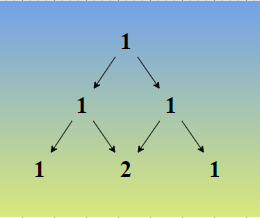The values of the last row give us the value of coefficients.

c0 = 1, c1 = 2, c2 =1

(a + b)2 = a2b0 + 2a1b1 + a0b2

Thus verified.

Example 3: Expand using Pascal Triangle (a + b)6

Solution:

First write the generic expressions without the coefficients.

(a + b)6 = c0a6b0 + c1a5b1 + c2a4b2 + c3a3b3 + c4a2b4 + c5a1b5 + c6a0b

Now let’s build a Pascal’s triangle for 7 rows to find out the coefficients.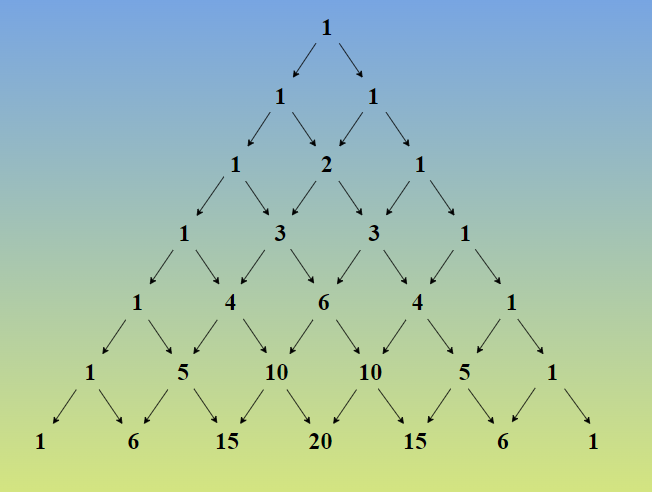The values of the last row give us the value of coefficients.

c0 = 1, c1 = 6, c2 = 15, c3 = 20, c4 =15, c5 = 6 and c6 = 1.

(a + b)6 = 1a6b0 + 6a5b1 + 15a4b2 + 20a3b3 + 15a2b4 + 6a1b5 + 1a0b

Example 4: Find the second element in the third row of Pascal’s triangle.

Solution:

We have to find the 2nd element in the 3rd row of Pascal’s triangle.

We know that the nth row of Pascal’s triangle is nC0, nC1, nC2, nC3…

The Pascal Triangle Formula is,

nCk = n-1Ck-1  + n-1Ck

where nCk represent (k+1)th element in nth row.

Thus, 2nd element in the 3rd row is,

3C1 = 2C0 + 2C1

= 1 + 2

= 3

Thus, the second element in the third row of Pascal’s triangle is 3.

Example 5: A coin is tossed four times, find the probability of getting exactly 2 tails.

Solution:

Using Pascal Triangle Formula,

Total number of outcomes = 2 = 16 (1 + 4 + 6 + 4 + 1 = 16)

Here we get four cases in which we get 2 tails,

Thus,

Probability of getting Two Tails = Favorable Outcome/Total Outcome

= 4/16 = 1/4

So the probability of getting exactly two tails is 1/4 or 25%

## FAQs on Pascal’s Triangle

### Q1: What is Pascal’s Triangle?

The triangle array of the number proposed by famous mathematician Balise Pascal is called Pascal’s Triangle. This triangle begins with 1 and in the next line the starting and the end numbers are fixed to be 1 then the middle number is generated by taking the sum of the above two numbers.

### Q2: What are the Uses of Pascal’s Triangle?

Pascal’s triangles have various uses,

• It is used to find the binomial coefficient of the binomial expansion.
• It provides an alternate way for expanding the binomial terms.
• It is used in algebra, probability theory, permutation and combination and other branches of mathematics.

### Q3: What is the Use of Pascal’s Triangle in Binomial Expansion?

We use Pascal’s triangle to easily find the coefficient of any term in the Binomial Expansion. Any row of Pascal’s Triangle (say nth) represents the coefficient of the Binomial expansion of the (x+y)n. For example, the second row of Pascal’s Triangle is, 1 2 1 and the expansion of (x+y)2

(x+y)2 = x2 + 2xy + y2

Here, the coefficient of each term is 1 2 1 which resembles the 2nd row of Pascal’s Triangle.

### Q4: What are the Various Patterns Found in Pascal’s Triangle?

Various patterns which we easily found in Pascal’s triangle are:

• Triangular Pattern
• Odd and even pattern
• Fibonacci pattern
• Symmetrical pattern

### Q5: What is the 5th Row of Pascal’s triangle?

The fifth row of Pascal’s triangle is represented below,

1 5 10 10 5 1

We know that sum of all the elements in any row is given using 2n where n represents the number of rows. Thus the sum of all the terms in the 5th row is,

25 = 32